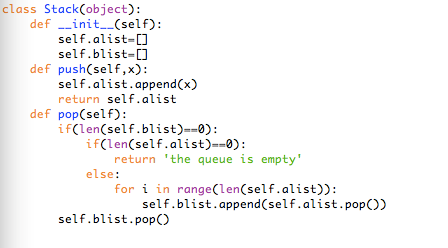今日头条面试经验

今日头条面试经验

（我选的python语言）上面是我昨天面试写的代码。整体来讲就是用两个list来模拟堆栈，命名为alist，blist。push操作时，将数据存到alist里。Pop操作时，从blist里面pop。如果blist里为空，就把alist的数据全部转移到blist里。如果alist也为空，就pop失败。但是只有在blist为空时，才可以从a往里转移数据，不然就不能保证输出数据有队列的性质了。我也不知道怎么解释，稍微画画图模拟一下就很简单啦。
（代码还有漏洞，只希望能加深你们对这题的理解，望不吐槽代码小渣渣。）
（然后就觉得面试官对这题的答案贼满意，大概是挽回了前两题写不出代码的损失）

第二轮：先自我介绍了一下，然后什么也没问，直接开始写代码，不过感觉这个人主要关注了我的算法思想，第二题代码写了一半，有一个框架就没让我继续写了。（半个小时）

第三轮：好像闯到了最后一关，到了主管面试环节，主管超级严肃，进来问了下什么时候可以入职，什么时候工作，一些很随意的问题，问了简历上两个项目，就又开始写代码。。。。。就一道题。（半个小时）

Hr面就很随意啦。我的hr也是一枚实习生，刚入职一个月。

package solutions._40;

import java.util.ArrayList;
import java.util.Arrays;
import java.util.List;

/**
* 40. Combination Sum II
*/

class Solution {
private boolean[] visited;
private List<List<Integer>> result = new ArrayList<>();
private List<Integer> cur = new ArrayList<>();

private void DFS(int[] arr, int target, int pos, int sum) {
if (sum > target) {
return;
}
if (target == sum) {
return;
}

for (int i = pos; i < arr.length; i++) {
if (visited[i]) {
continue;
}
if (i != 0 && arr[i] == arr[i - 1] && !visited[i - 1]) {
continue;
}
if (!visited[i]) {
visited[i] = true;
DFS(arr, target, i, sum + arr[i]);
visited[i] = false;
cur.remove(cur.size() - 1);
}
}
}

public List<List<Integer>> combinationSum2(int[] candidates, int target) {
Arrays.sort(candidates);
visited = new boolean[candidates.length];
DFS(candidates, target, 0, 0);
return result;
}

public static void main(String[] args) {
Solution solution = new Solution();
int[] arr = {10, 1, 2, 7, 6, 1, 5};
List<List<Integer>> list = solution.combinationSum2(arr, 8);
System.out.println(list);
}
}
package solutions._39;

import java.util.ArrayList;
import java.util.List;

/**
* 39. Combination Sum
*/
class Solution {
private List<List<Integer>> result = new ArrayList<>();

private void DFS(int[] arr, int pos, int curSum, int sum) {
if (curSum > sum) {
return;
}

if (curSum == sum) {
return;
}

for (int i = pos; i < arr.length; i++) {
DFS(arr, i, curSum + arr[i], sum);
cur.removeLast();
}
}

public List<List<Integer>> combinationSum(int[] candidates, int target) {
DFS(candidates, 0, 0, target);
return result;
}

public static void main(String[] args) {
Solution solution = new Solution();
int[] arr = {2, 3, 6, 7};
int target = 7;
List<List<Integer>> list = solution.combinationSum(arr, target);
System.out.println(list);
}
}

1.3

import java.util.Stack;

public class Solution {
Stack<Integer> stack1 = new Stack<Integer>();
Stack<Integer> stack2 = new Stack<Integer>();

public void push(int node) {
stack1.push(node);
}

public int pop() {
if (!stack2.isEmpty()) {
return stack2.pop();
}
while (!stack1.isEmpty()) {
stack2.push(stack1.pop());
}
return stack2.pop();
}
}

2.1

2.2

[0,1,2,4,5,6,7]
[1,2,4,5,6,7,0]
[2,4,5,6,7,0,1]
[4,5,6,7,0,1,2]
[5,6,7,0,1,2,4]
[6,7,0,1,2,4,5]
[7,0,1,2,4,5,6]

• 一个元素就是它本身
• 两个元素的旋转数组可以变成递减的

• 划分为两个有序的子数组{4,5} {1,2,3}。
• 且第一个有序数组 大于等于 第二个有序数组。
• 且最小的数(1)刚好为这两个有序子数组的分割点。
• 第一个数(4)必然大于等于最后一个数(3)，否则这个旋转数组必然是有序的
/**
* 153. Find Minimum in Rotated Sorted Array
*/
class Solution {
public int findMin1(int[] nums) {
int i;
for (i = 1; i < nums.length; i++) {
if (nums[i] < nums[i - 1]) {
break;
}
}
if (i == nums.length) {
return nums;
}
return nums[i];
}

public int findMin2(int[] nums) {
int left = 0;
int right = nums.length - 1;
while (left < right) {
if (nums[right] > nums[left]) {
return nums[left];
}
int mid = left + (right - left) / 2;
if (nums[mid] >= nums[left]) {
left = mid + 1;
} else {
right = mid;
}
}
return nums[right];
}

public static void main(String[] args) {
Solution solution = new Solution();
int[] arr = {4, 5};
System.out.println(solution.findMin1(arr));
System.out.println(solution.findMin2(arr));
}
}